Eighth Mississippi State - UAB Conference on Differential Equations and Computational Simulations. Electron. J. Diff. Eqns., Conference 19 (2010), pp. 245-255.

### Bifurcation of solutions of separable parameterized equations into lines Yun-Qiu Shen, Tjalling J. Ypma

Abstract:
Many applications give rise to separable parameterized equations of the form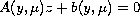, where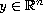,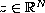and the parameter; here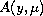is an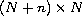matrix and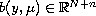. Under the assumption thathas full rank we showed in  that bifurcation points can be located by solving a reduced equation of the form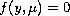. In this paper we extend that method to the case thathas rank deficiency one at the bifurcation point. At such a point the solution curve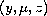branches into infinitely many additional solutions, which form a straight line. A numerical method for reducing the problem to a smaller space and locating such a bifurcation point is given. Applications to equilibrium solutions of nonlinear ordinary equations and solutions of discretized partial differential equations are provided.

Published September 25, 2010.
Math Subject Classifications: 65P30, 65H10, 34C23, 37G10.
Key Words: Separable parameterized equations; bifurcation; rank deficiency; Golub-Pereyra variable projection method; bordered matrix; singular value decomposition; Newton's method.

Show me the PDF file (248K), TEX file, and other files for this article.Yun-Qiu Shen Department of Mathematics, Western Washington University Bellingham, WA 98225-9063, USA email: yunqiu.shen@wwu.edu Tjalling J. Ypma Department of Mathematics, Western Washington University Bellingham, WA 98225-9063, USA email: tjalling.ypma@wwu.edu